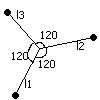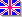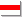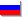### Three-end string

Three-end string is the most simple example of non-trivial topology, so-called bundle, and it can be considered as the mechanical analogy of much more complicated physical systems with non-trivial topological structures. Such models are often used in different areas of theoretical physics (physics of elementary particles, quantum theory of gravitation and so on). The ends of three strings are conjoined as shown below.In the string with one fixed point waves are described by such functions:
f(x,t)=A(Sin(wt-kx) - Sin(wt+kx))  or
f(x,t)=A*Cos(wt + q)Sin(kx)   (*)
To apply this to our model of the three-end string we have to assume some supplementary conditions on the conjoined ends of three strings. These conditions, are, naturally, equalities of amplitudes and smoothness of the resulting functions in the point of junction:
f(l1,t) = f(l2,t) = f(l3,t)
where l1, l2, l3 are the length of each string.
df(x1,t)/dx1 + df(x2,t)/dx2 + df(x3,t)/dx3 = 0
or, using (*):
A1Sin(kl1) = A2Sin(kl2) = A3Sin(kl3)
A1Cos(kl1) + A2Cos(kl2) + A3Cos(kl3) = 0
From these equations we can obtain fundamental wavenumbers of our system. They are the solutions of this equation, which is the consequence of previous two equations:
Sin(kl1)Sin(kl2)Cos(kl3) + Sin(kl3)Sin(kl1)Cos(kl2) + Sin(kl2)Sin(kl3)Cos(kl1) = 0
For l1 = l2 = l3 = l :
3*Sin2(kl)Cos(kl) = 0. Therefore
kn = pi*n/l, kn = pi*(n + 1/2)/l

Here is the Delphi project with real-time emulator of three-end string. If your computer is too fast;)) you can correct FPS constants in form unit.
String.zip

©2002-2003, VeterEnglishБеларускаяРусский
Сайт создан в системе uCoz